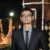Disclosure: This post may contain affiliate links, meaning when you click the links and make a purchase, we receive a commission.

# How to Multiply Columns, Cells, Rows, & Numbers in Excel

Multiplying numbers in excel is very easy. But while multiplying multiple cells, columns and rows, you often face difficulties. Knowing different methods of multiplication in excel may save your calculation time. In this tutorial, we will see how to multiply columns, cells, rows, and numbers in Excel in more than one way.

## How to multiply numbers in Excel

Multiplying numbers is the easiest method of multiplication. It is just like using a calculator. You just need to use “=” and “*” signs with the numbers. The following example shows us how multiplication of numbers is done in excel.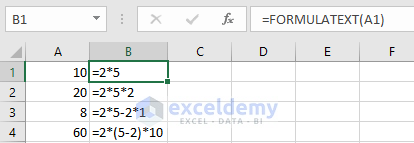Note: Here to show you the formulas that have been used in this example I used a function named “FORMULATEXT”. After writing the FORMULATEXT it will ask for the reference. Just insert the reference cell and it will show you what formula has been used.

## How to multiply cells in Excel

Instead of numbers, we actually work with cells in excel. So, knowing the multiplication method of different cells is important. Here instead of numbers that we used in the previous example, we will be working with cells.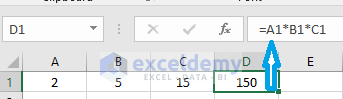In the above example, the numbers 2,5 and 15 are in the cells of A1, B1, and C1 respectively. To multiply this numbers, we can simply use the formula =A1*B1*C1. In this way, we can multiply countless numbers.

## How to multiply columns in Excel

### Dragging formula in excel

Here we will be using a table of 3 columns. The 1st column consists of the product name. The rest 2 columns consist Unit price and Quantity. We will be multiplying the Unit price with Quantity, which means we are will actually multiply column B and D. For multiplying these two columns in excel, 1st write the multiplication formula for the topmost cell, for example, =B2*C2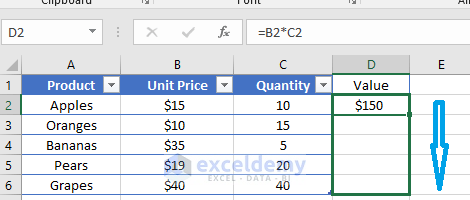It will show the value of 15*10 which is 150. By dragging the formulated cell which is D2 in the downwards we can copy the formula for the rest of the D column. This will carry out the multiplicated result of column B and column C in column D.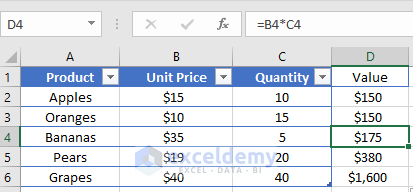We can also apply the multiplication formula for multiple columns. In that case, only the cells number will increase.

### Array multiplication in Excel (for Columns)

There is another way of multiplying between columns. It is done by making an array of columns. Instead of using a single cell for applying the formula, we will be indicating the whole range where the formula will be applied. The procedure is given below.

• 1st Indicate the entire range where you want to apply your formula. In this case, the range is D2: D6
• Type the formula =B2:B6*C2: C6 in the formula bar. Then press Ctrl + Shift + Enter. Excel will enclose the formula in curly brace {} which indicates an array formula. You will be able to see the result in the whole column D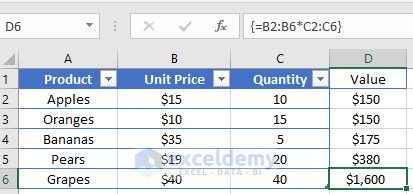Note: If you want to put the curly braces manually the formula won’t work at all.

## How to multiply rows in Excel

### Dragging formula in Excel

Multiplying between rows is not a regular task in excel. The procedure is same as multiplying between columns. 1st insert the multiplication formula in the leftmost cell. For example, =B1*B2. It will show the value 50 as 5*10=50.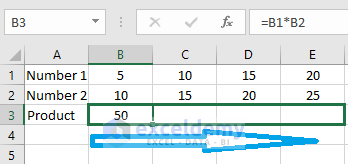By dragging the formulated cell which is B3 in the right-side we can copy the formula for the rest of Row 3. This will carry out the multiplicated result of row 1 and row 2 in row 3.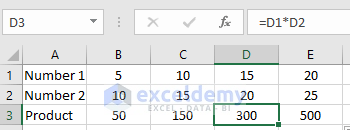Applying the multiplication formula for multiple rows will be same. The only added thing in the formula will be the increasing number of cells.

### Array multiplication in Excel (For Rows)

Again, the array multiplication method which we used for columns multiplication can also be used for rows multiplication. The following picture shows the result.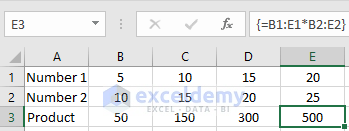Here the formula that was applied is =B1:E1*B2: E2 and the range in which the result was shown is B3: E3.

## How to use PRODUCT function in Excel

To multiply cells or ranges in excel we can use the PRODUCT function instead of using multiplication symbol. The product function is the fastest way of multiplication between ranges. To multiply values in cells A1 and B1 use this formula:
=PRODUCT(A1, B1)

Similarly, to multiple values in cells A2, B2, and C2 use this formula:
=PRODUCT (A2: C2)
The term A2: C2 indicates A2 through C2.

We can also use the product formula while multiplying a range of cells with a random number. Let’s say we want to multiply the numbers from cell A2 through C2 with a random number 5. The formula will be =PRODUCT (A2: C2,5)

The following picture shows these 3 examples in one frame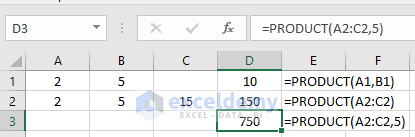## How to multiply a column by a number in Excel

Let’s say we want to calculate the area of some rectangles where the length is fixed to 10 meters and we have variable widths of the rectangles. The widths are in one column and the length is in a fixed cell. The formula for calculating the Area is Length*Width. Here we will actually multiply a column by a number/cell having a fixed value to calculate the area of rectangles. Let’s start with the uppermost cell in column D. here we use the formula =C2*\$A\$2. The \$ sign is used for locking the reference.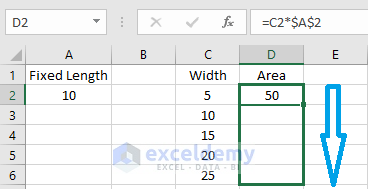By dragging the formulated cell, we will see the result for the rest of the cells of column D.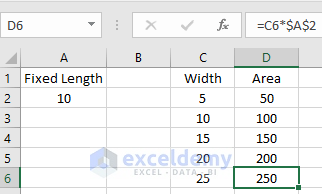Note: For locking of the reference cell/number \$ sign is mandatory. The normal multiplication with dragging method won’t give us the proper result. The following picture shows us the result without using the \$ sign which is not accurate at all.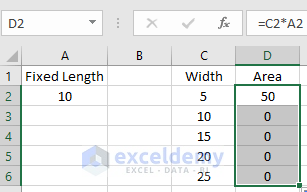## How to multiply percentages in Excel

Percentages can be multiplied by 3 methods in excel. These methods are given below.

• Multiplying the number by percentage: =25*15%
• Multiplying the number by percentage equivalent number: =25*0.15 (as 15%=0.15)
• Multiplying the cell by percentage: =A2*15%

The following picture shows all these three methods in one frame.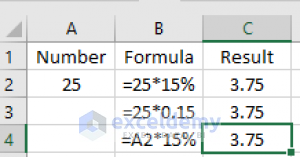## How to use Paste Special multiply in Excel

Paste special multiply feature is easier to use when you have to multiply a single number with a column/row of numbers. In the previous example locking the cell reference was necessary. Here no locking is required. The procedure is simple.

• First copy the numbers of the column you want to multiply by the single number (Here it is C2: C6)
• Paste the numbers in the column where you want to show your result (D2: D6 is used)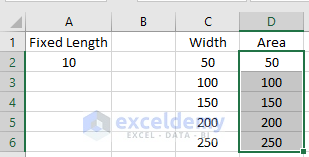• Then copy the single number that you want to multiply with the column of numbers. (The number is located in A2)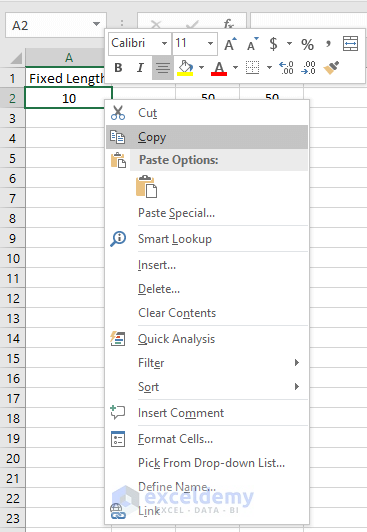• Select the range where you want to multiply the number then instead of pasting it click on the Paste Special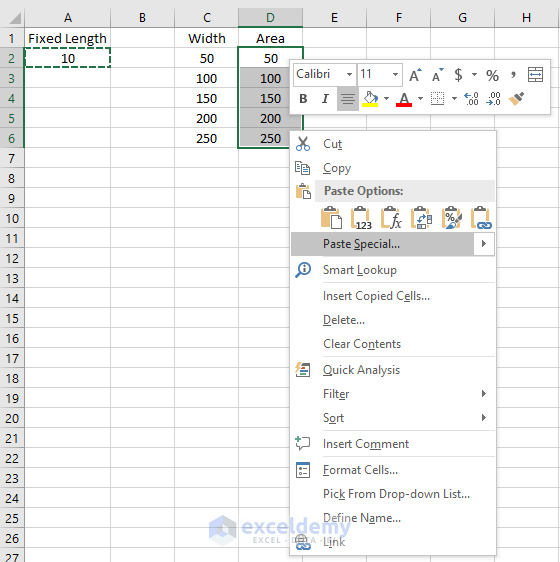• A box titled Paste Special will come up. Tick on the Multiply box and press OK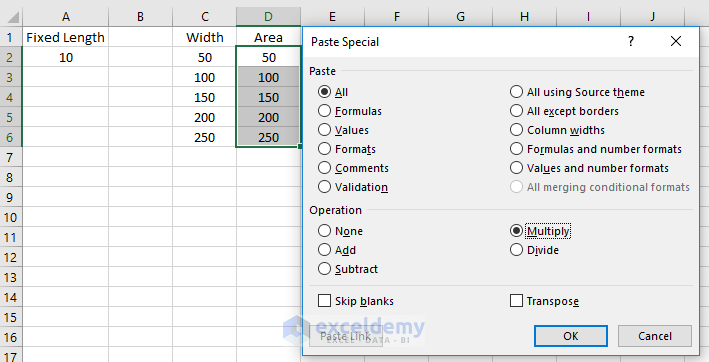• You will find out your desired result in the specified column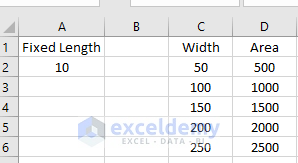## SUMPRODUCT function in Excel

Suppose you have different fruits with unit price and quantity. You sold them all, and now you want to calculate the total sales. In this kind of calculation, you can directly use the SUMPRODUCT function rather doing a manual calculation. In the manual calculation, you have to multiply every unit price with quantity and after this, you need to add up the subtotal.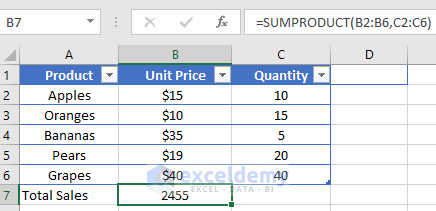## Array multiplication in Excel

Finding the average, total, Maximum and minimum between the product of two or multiple columns require some array multiplication. It’s not a difficult task to perform. Here, in this kind of problem-solving task, we require some functions of Microsoft Excel. The following picture shows the array multiplication example with different functions.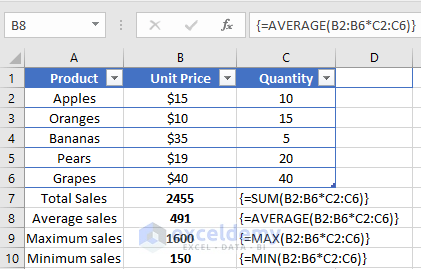For completing the array formula properly be sure to press Ctrl + Shift + Enter instead of pressing Enter. By doing this, excel will enclose the formulas in curly braces {}.

## Conclusion

As we can see multiplication in excel is not a difficult task. You either use the multiplication symbol or the Product function to multiply between cells, columns, or rows. But you have to be tricky enough to judge how you are going to approach. This article may help you to find shortcuts to excel multiplication while doing the calculations effectively.

Hello!

Welcome to my Profile. Here I will be posting articles related to Microsoft Excel. I have completed my BSc in Electrical and Electronic Engineering from American International University-Bangladesh. I am a diligent, goal-oriented engineer with an immense thirst for knowledge and attitude to grow continuously. Continuous improvement and life-long learning is my motto.

1. Reply• Reply